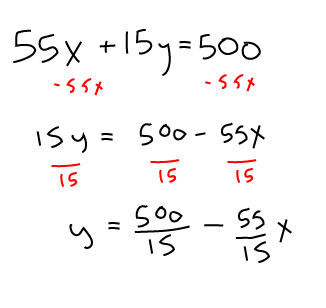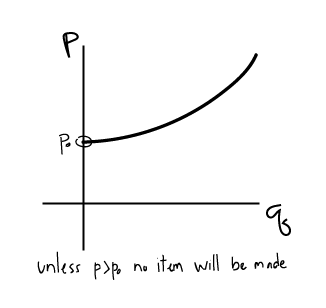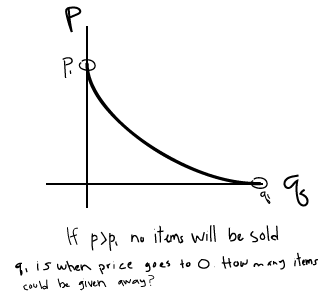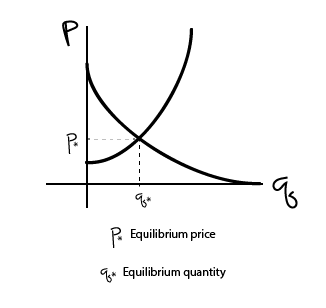blog home

# M119 Notes - Week 2 - Supply and Demand

Subject: Calculus 1
Courses: M119
Posted by: Sarah

## 1.4b Budget constraint problem with fixed prices for 2 items

You have a budget of 500 dollars for books ($x$) and CDs ($y$). The total spent on books is $55x$ and the total spent on CDs is $15y$. Write an equation in the form of $y = mx+b$ then solve for $y$.## Supply and demand problems

Supply and demand both relate quantity $q$ of an item to the price $p$.

### Supply curve: Increasing function. $q$ increases as $p$ increases

• The higher the price that sellers can charge for an item, the more quantity they will produce.
• If sellers can NOT sell an item for more money than it costs to manufacture, then they would choose not to manufacture it.### Demand Curve: Decreasing function. $q$ decreases as $p$ increases

• The more an item costs, the less buyers will want to buy it (less quantity will be demanded).
• If price is too high, NO items will be sold.### Equilibrium point: It is assumed that the market settles to an equilibrium point when $S(p) = D(p)$.

• The supply and demand curves intersect.
• Corresponds to a specific price and quantity.## EXAMPLE

Find the equilibrium $p$ and $q$ for the following supply and demand functions:
$S(p) = 10p - 500$
$D(p) = 2500 - 20p$

1. Set $S(p)$ equal to $D(p)$.

$10p - 500 = 2500 - 20p$
2. Solve for $p$.

$30p = 3000$
$p = 100$
3. To solve for $q$, notice the $S(p)$ or the supply at any given price is actually $q$ or the quantity.

$q = S(p) = 10p - 500$
4. Plug in the value that you got for $p$ into that equation.

$q = 10*100 - 500$
$q= 500$

## Taxes

• Two kinds:
• Tax on consumers: sales tax, affects demand curve.
• Tax on producers: affects supply curve.
• How taxes affect supply and demand curves:
• For a tax of $x$ dollars, supply curve is $S(p-x)$.
• For a tax of $x$ dollars, demand curve is $D(p+x)$.
• For a tax of $x\%$, supply curve is $S(p-px)$.
• For a tax of $x\%$, demand curve is $D(p+px)$.
• Taxes will affect equilibrium.

### How do taxes affect suppliers and producers?

The price of an item was 100 dollars and a tax of 6 dollars is imposed. The price is now 102 dollars. How much tax is paid by the consumer? How much is paid by the producers?